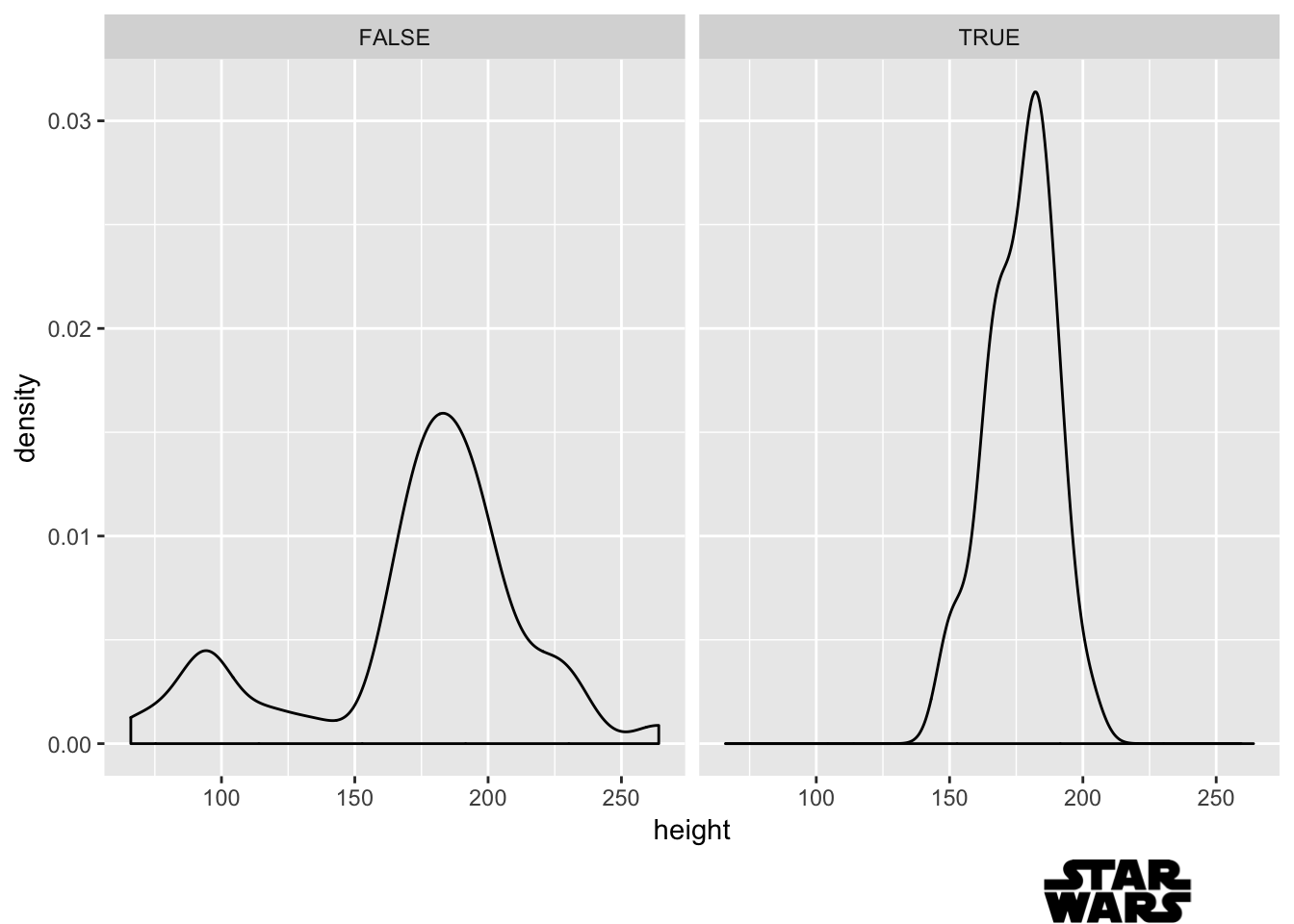How I Put Logos on ggplot2 Figures

Creating a ggplot2 theme that matches your organization’s colors and fonts can help your plots look slick and feel seamless with the rest of the organization’s work. One extra thing that has come up with this for me has been adding a logo to plots. While I find customizing the theme by using theme() to be pretty straightforward, I feel like adding a logo is a little trickier. So in this post, I show how I add logos to ggplot2 figures. The code is cobbled from other blog posts and StackOverflow questions, but I wanted to put it all in one place and show what was most intuitive for me.

First, let’s make a plot to add a logo to. I use the starwars data set, which is included in the dplyr package—loaded below with library(tidyverse). We can look at what species are represented more than once in this data set:

library(tidyverse)
data("starwars")

(species <- starwars %>%
count(species) %>%
filter(!is.na(species) & n > 1) %>%
arrange(-n) %>%
mutate(species = factor(species, species)))
## # A tibble: 8 x 2
##   species      n
##   <fct>    <int>
## 1 Human       35
## 2 Droid        5
## 3 Gungan       3
## 4 Kaminoan     2
## 5 Mirialan     2
## 6 Twi'lek      2
## 7 Wookiee      2
## 8 Zabrak       2

Then we plot these counts:

(p1 <- ggplot(species, aes(x = species, y = n)) +
geom_bar(stat = "identity") +
theme_light())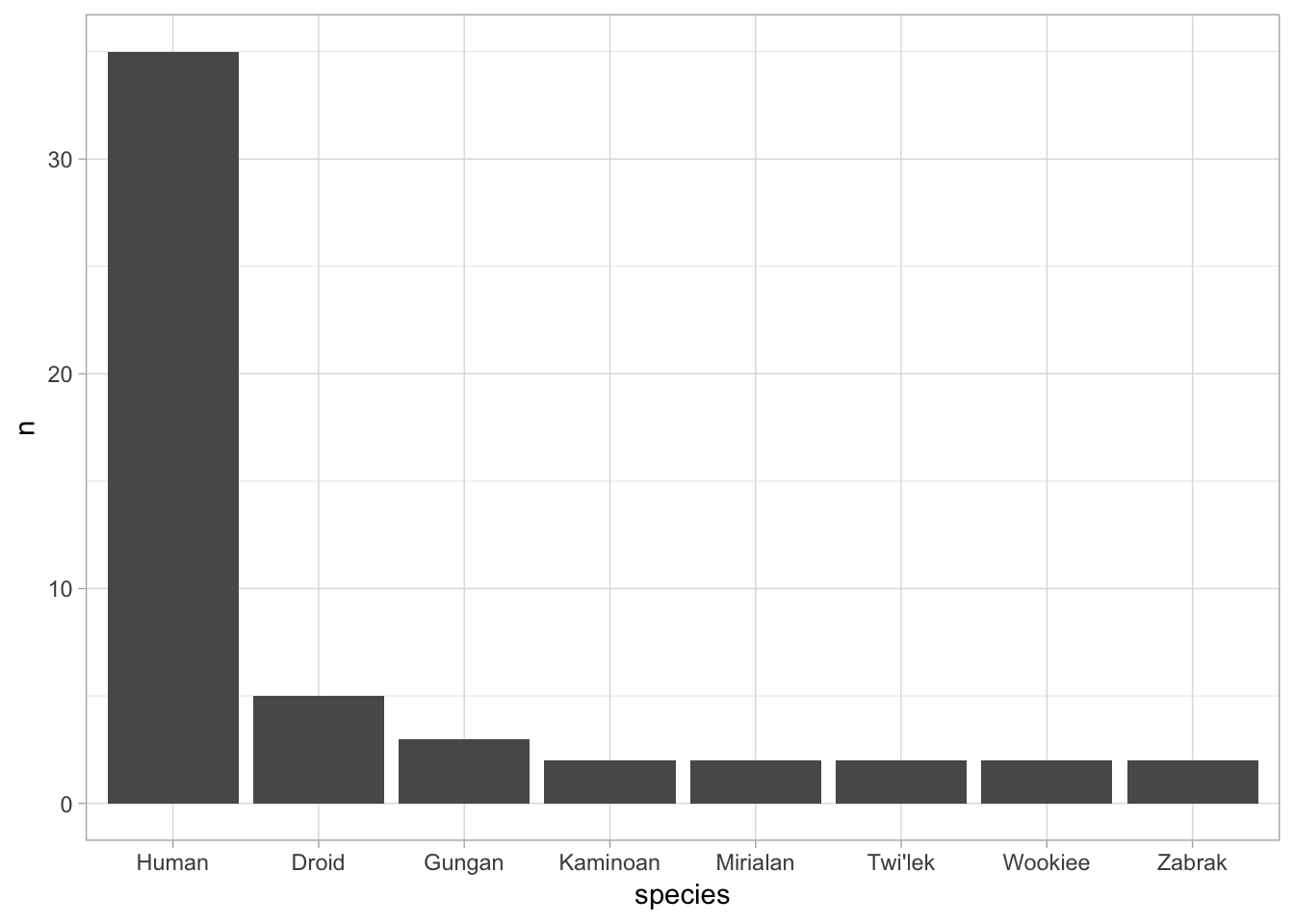Now, for a logo. I will be working with .png files in this post, and I have a file stored in my working directory called logo.png. The following code can take a file name for a .png and return an object that ggplot2 can use:

get_png <- function(filename) {
grid::rasterGrob(png::readPNG(filename), interpolate = TRUE)
}

l <- get_png("logo.png")

Now we have our logo as the object l. I like to stick logos below the plot and to the right. We do this in three steps:

• annotation_custom: This places the logo in a specific range of the plot. We specify four points that draw a container around where we want to place the logo. Note that these numbers follow the same scale as your data.

• coord_cartesian: We use this to turn the clip off so that the plot isn’t cropped down to only include where data are. This can be used any time we want to do something in the margins.

• theme: We specify the plot margins. The units follow the pattern top, right, bottom left (remember: trbl or “trouble”). In the code below, I specify a larger padding on the third position (i.e., below) so that we have some white space to work in for the logo.

I like to use grid::roundrectGrob() as a test logo when I’m trying to figure out the correct four points to supply to annotation_custom. This will just draw a rectangle for the container that your logo will be placed inside of. I assign that to t as a test logo:

t <- grid::roundrectGrob()

p1 +
annotation_custom(t, xmin = 6.5, xmax = 8.5, ymin = -5, ymax = -8.5) +
coord_cartesian(clip = "off") +
theme(plot.margin = unit(c(1, 1, 3, 1), "lines"))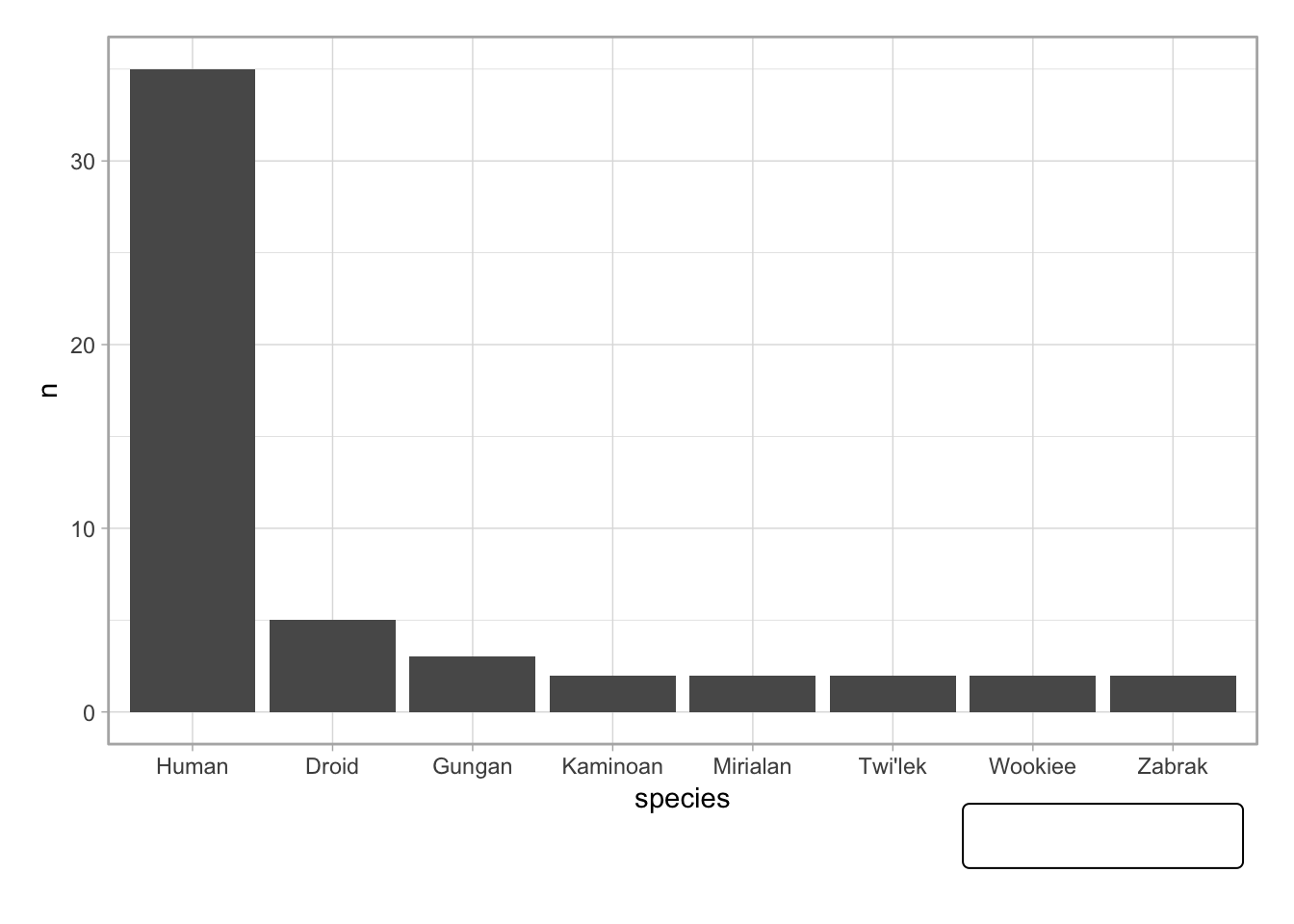Now that I know this is the correct placement, I swap out t for l:

p1 +
annotation_custom(l, xmin = 6.5, xmax = 8.5, ymin = -5, ymax = -8.5) +
coord_cartesian(clip = "off") +
theme(plot.margin = unit(c(1, 1, 3, 1), "lines"))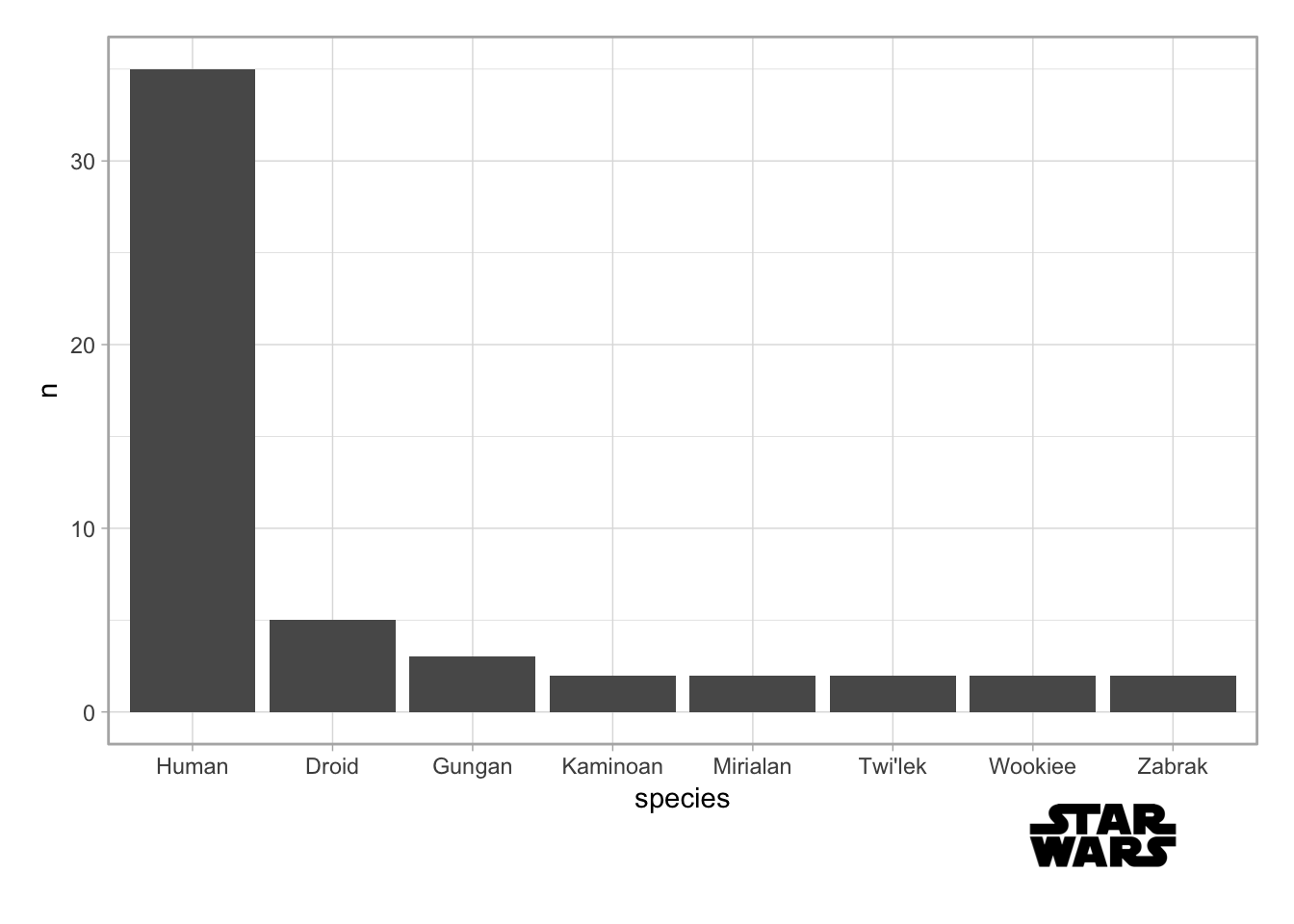And we have a logo placed right under the plot!

One issue I run into using this approach is whenever we want to use facet_wrap() or facet_grid(). Using this approach will try to add the logo at the bottom of every panel:

p2 <- starwars %>%
mutate(human = !is.na(species) & species == "Human") %>%
ggplot(aes(x = height)) +
geom_density() +
facet_wrap(~ human)

p2 +
annotation_custom(t, xmin = 200, xmax = 275, ymin = -.005, ymax = -.008) +
coord_cartesian(clip = "off") +
theme(plot.margin = unit(c(1, 1, 3, 1), "lines"))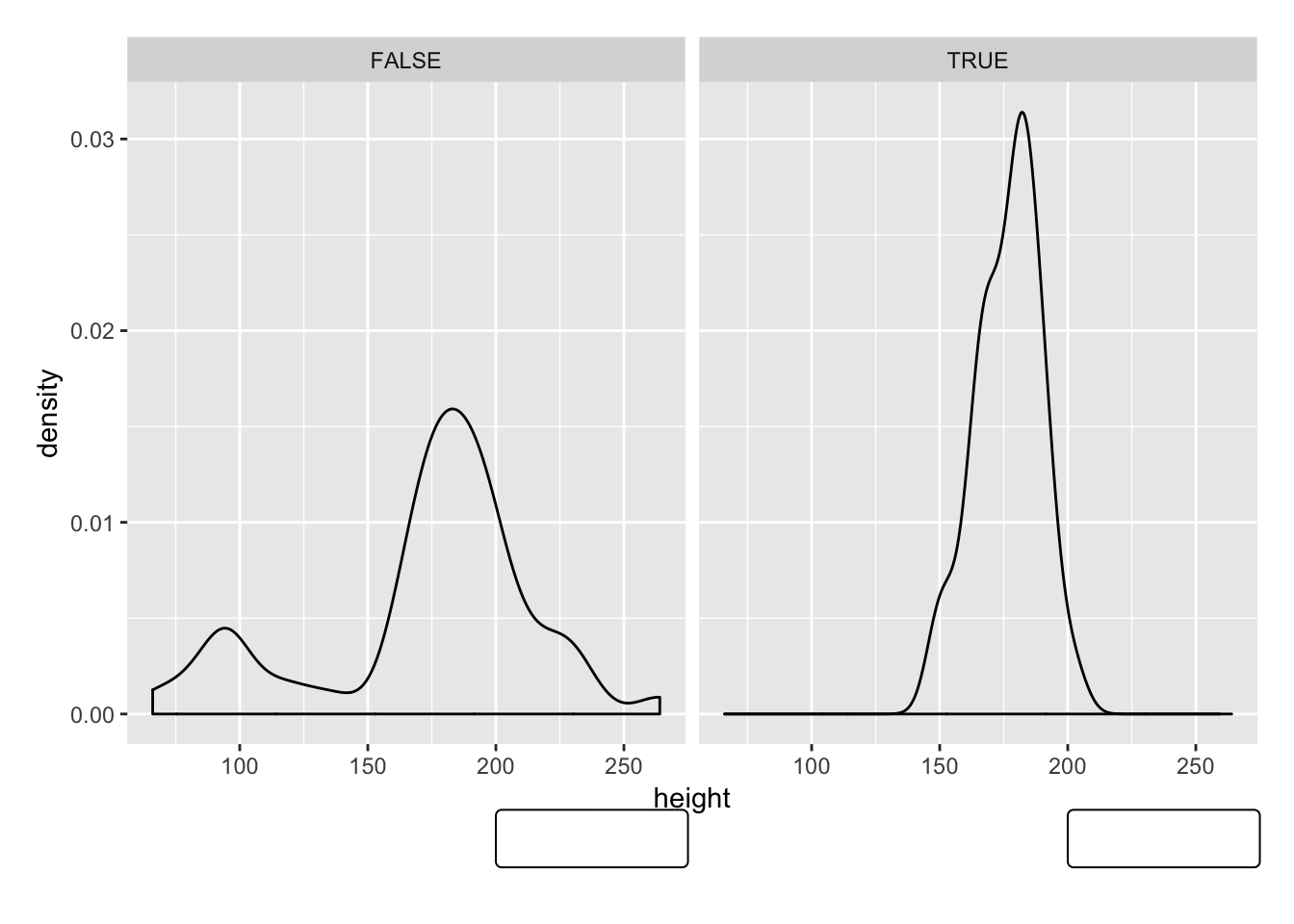So, what I do instead is create a plot that is only the logo. I make the x-axis data be the vector 0:1. This way, I can specify putting the logo on .80 to 1.0 if I want to get the right-most 20% of the figure. I make the y-axis data be the integer 1; I don’t specify ymin or ymax so that the logo will fill this entire height of the plot. I also use theme_void() to get rid of anything else but the logo.

(p3 <- ggplot(mapping = aes(x = 0:1, y = 1)) +
theme_void() +
annotation_custom(l, xmin = .8, xmax = 1))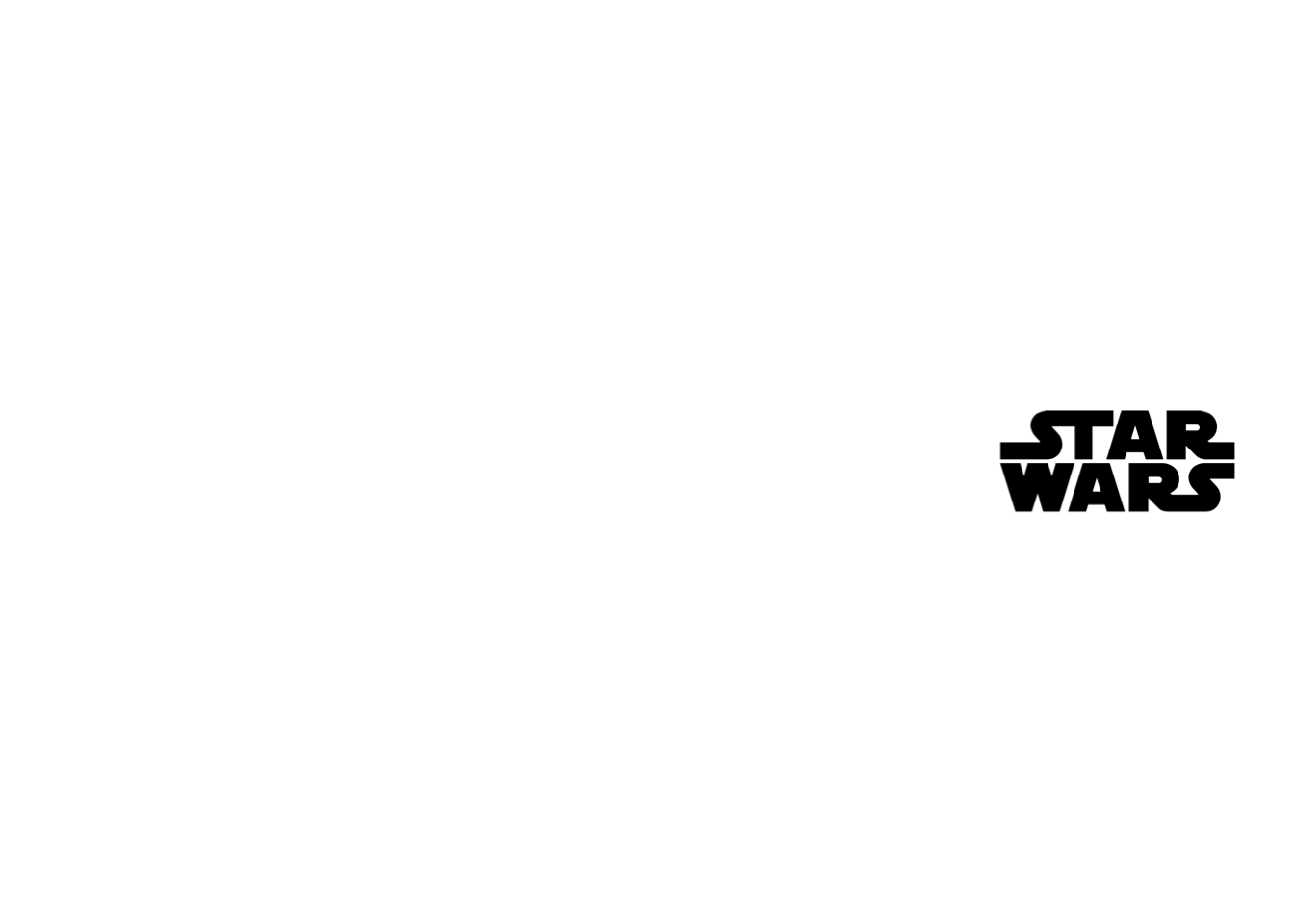Then, I use gridExtra::grid.arrange() to stack the main plot itself on top of the logo plot. The heights argument means that p2 is 93% of the height, and p3 is 7%:

gridExtra::grid.arrange(p2, p3, heights = c(.93, .07))